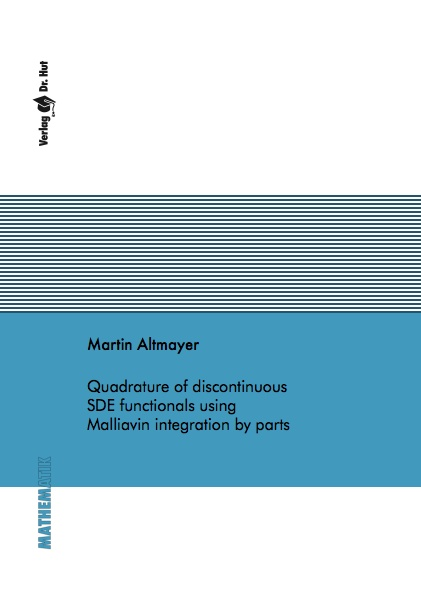Datenbestand vom 20. August 2019aktualisiert am 20. August 2019

# ISBN 9783843922388Euro 72,00 inkl. 7% MwSt

978-3-8439-2238-8, Reihe Mathematik

Martin Altmayer
Quadrature of discontinuous SDE functionals using Malliavin integration by parts

106 Seiten, Dissertation Universität Mannheim (2015), Hardcover, A5

## Zusammenfassung / Abstract

One of the major problems in mathematical finance is the pricing of options. This requires the computation of expectations of the form E(f(S_T)) with S_T being the solution to a stochastic differential equation at a specific time T and f being the payoff function of the option. A very popular choice for S is the Heston model.

While in the one-dimensional case E(f(S_T)) can often be computed using methods based on PDEs or the FFT, multidimensional models typically require the use of Monte-Carlo methods. Here, the multilevel Monte-Carlo algorithm provides considerably better performance - a benefit that is however reduced if the function f is discontinuous. This thesis introduces an approach based on the integration by parts formula from Malliavin calculus to overcome this problem: The original function is replaced by a function containing its antiderivative and by a Malliavin weight term. We will prove that because the new functional is continuous, we can now apply multilevel Monte-Carlo to compute the value of the original expectation without performance reduction. This theoretical result is accompanied by numerical experiments which demonstrate that using the smoothed functional improves performance by a factor between 2 and 4.

Furthermore, the same integration by parts trick that was used to smooth the functional can be applied to derive a weak rate of convergence in the Heston model without making any smoothness assumptions on the payoff f - at the price of a rather strong condition on the model parameters.